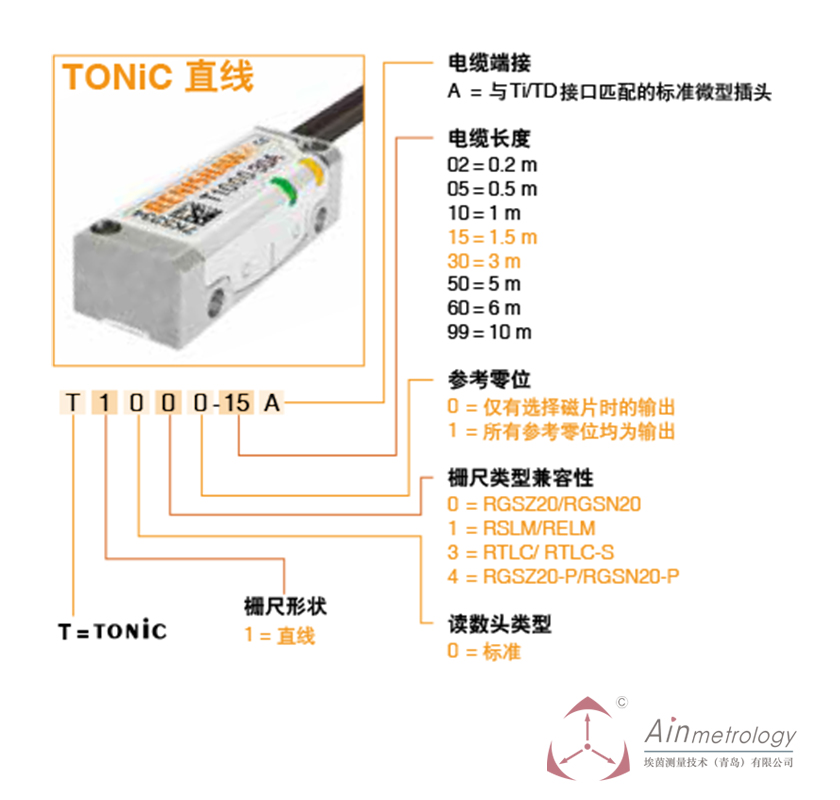•RENISHAW TONIC系列读数头及参数编号

# RENISHAW TONIC系列读数头及参数编号

RENISHAW TONIC 系列读数头

0 = RGSZ20/RGSN20
1 = RSLM/RELM
3 = RTLC/ RTLC-S
4 = RGSZ20-P/RGSN20-P

0 = 仅有选择磁片时的输出
1 = 所有参考零位均为输出
-
15代表线缆长度：
02 = 0.2 m
05 = 0.5 m
10 = 1 m
15 = 1.5 m
30 = 3 m
50 = 5 m
60 = 6 m
99 = 10 m
A代表电缆端接
A = 与Ti/TD接口匹配的标准微型插头